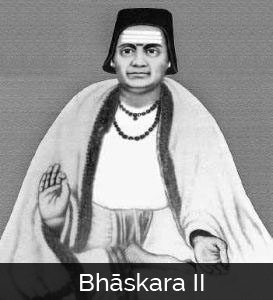# e-olymp 8514. Never drink too much!

The task is taken from e-olymp

# Task

Mahmoud together with his friends visited Georgia. They would stay in a hotel at Rustavelli. When the cowboys reached the hotel, they hung their hats in the entrance and settled in. The beer bottles on the table could not escape from Mahmoud’s attention when passing through the corridor. At the suggestion of Mahmoud, all the cowboys began drinking. They drank too much, thus none of them was mindful. Then they decided going downtown. On the way out, everyone had a hat on, but they mixed up the hats as they were so drunk.

The man who is able to have on his own hat while he is drunk is considered clever and who is not able to do so is considered stupid.

You are given the number of cowboys — n (including Mahmoud). You should find in how many ways the cowboys may have on the hats so that all of them are stupid. Two ways are considered different if there is at least one cowboy who has a hat in this case and another hat in the other case.

As the answer may become very large, you should output the result modulo 109 + 7.

# Input

Given the number of cowboys — n (1 ≤ n ≤ 107).

# Output

The answer to the problem as specified above.

# Tests

 № Inputs Outputs 1 1 0 2 4 9 3 9 133349 4 555 335132588 5 10000000 824182295

# Solution

We have all possible permutations $C_n^0 \cdot n!$ , minus one fixed (one of them is not stupid) we get $C_n^0 \cdot n!-C_n^1 \cdot (n-1)!$ but we subtract for minimum fixed pair (two of them are not stupid) we need to add them $C_n^0 \cdot n!-C_n^1 \cdot (n-1)! + C_n^2 \cdot (n-2)!$ etc.
So the $k$ member is $C_n^k \cdot (n-k)! \cdot (-1)^k$
Lets find the attitude of $k$ member to the previous one. Its $-k -1$
The last one will be $-1$ it depends on parity of $n$.
First two are the same ( $n!$ ) so we skip them, but in this case we need to check if$n$ equals $1$
And next we make a loop to find the answer by multiplying start value and add it to the answer.
The complexity is $O(n)$

# e-olymp 2062. Лилавати

Задача взята с сайта e-olymp

# ЗадачаКрупнейшему индийскому математику XII в. Бхаскаре принадлежит трактат «Сиддханта-широмани» («Венец учения»), переписанный в XIII в. на полосках пальмовых листьев. Этот трактат состоит из четырех частей, из которых «Лилавати» посвящена арифметике, «Биджаганита» — алгебре, остальные две части астрономические. «Лилавати» (что значит «прекрасная») Бхаскара посвятил своей дочери.

Многие свои задачки Бхаскара излагал в поэтической форме, вот одна из них:

Из множества чистейших цветков лотоса
Третья часть была принесена в дар Шиве,
Пятая часть – Вишну, шестая часть – Солнцу;
Четвёртую часть всех цветков получил Бхвани,
А оставшиеся шесть цветков были даны высокочтимому Учителю.

Мы не можем дословно передать всю прелесть и красоту звучания этих стихов Древней Индии, поэтому нашу задачку сформулируем в прозе. Итак, эта же задачка в общем виде: «В дар Шиве принесли A-ую часть цветков лотоса, в дар Вишну – B-ую часть, в дар Солнцу – C-ую часть, для Бхвани досталась D-ая часть и высокочтимый Учитель получил E цветков. Сколько всего цветков лотоса было в распоряжении дарившего?»

# Входные данные

В первой и единственной строке входных данных заданы через пробел 5 неотрицательных целых чисел: A, B, C, D и E, каждое из которых не превышает 100.

# Выходные данные

Вывести единственное число – ответ на задачу, или –1 в случае, если входные данные противоречивы, либо же решить задачку однозначно не предоставляется возможным.

# Тесты

# Входные данные Выходные данные
1 3 5 6 4 6 120
2 8 8 0 4 20 40
3 2 10 20 0 1 -1
4 2 3 20 60 1 -1
5 2 4 8 16 1 16

# Решение

Целое это 1, поэтому $1-(\frac{1}{a}+\frac{1}{b}+\frac{1}{c}+\frac{1}{d})=\frac{1}{e}$

В свою очередь общее количество цветков будет $e\cdot(\frac{a\cdot b\cdot c\cdot d}{a\cdot b\cdot c\cdot d-(b\cdot c\cdot d+a\cdot c\cdot d+a\cdot b\cdot d+a\cdot b\cdot c)})$

Остается учесть что входные данные могут иметь нули. И в конце проверить чтоб количество цветов принесённых в дар было целым числом для каждого из Богов.